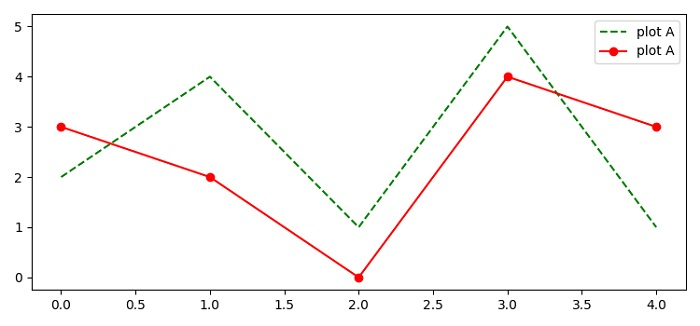# How to name different lines in the same plot of Matplotlib?

To name different lines in the same plot of matplotlib, we can take the following steps −

• Set the figure size and adjust the padding between and around the subplots.

• Make two lists of data points.

• Plot point1 and point2 using plot() method.

• Place a legend on the figure.

• To display the figure, use show() method.

## Example

import matplotlib.pyplot as plt
plt.rcParams["figure.figsize"] = [7.50, 3.50]
plt.rcParams["figure.autolayout"] = True

points1 = [2, 4, 1, 5, 1]
points2 = [3, 2, 0, 4, 3]

plt.plot(points1, 'g--', label="plot A")
plt.plot(points2, 'r-o', label="plot A")
plt.legend()

plt.show()

## Output